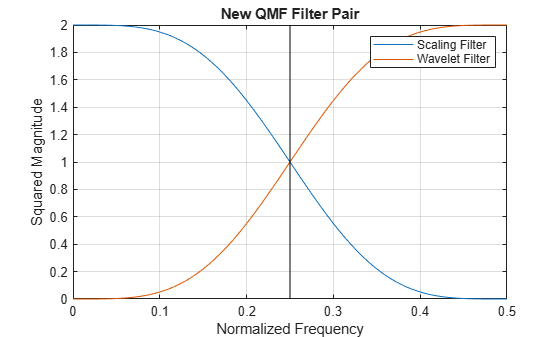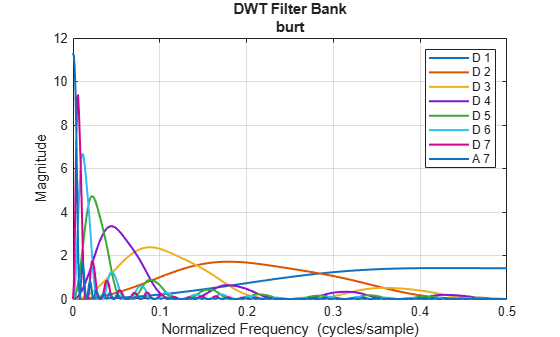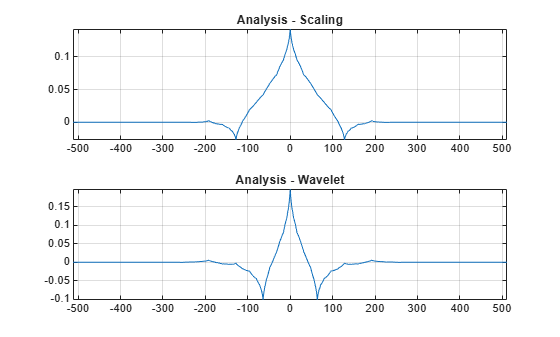This example shows how to add an orthogonal quadrature mirror filter (QMF) pair and biorthogonal wavelet filter quadruple to Wavelet Toolbox™. While Wavelet Toolbox™ already contains many orthogonal and biorthogonal wavelet families, you can easily add your own filters and use the filter in any of the discrete wavelet or wavelet packet algorithms.

This section shows how to add a QMF filter pair. Because the example is only for purposes of illustration, you will add a QMF pair that corresponds to a wavelet already available in Wavelet Toolbox™.

Create a vector `wavx` that contains the scaling filter coefficients for the Daubechies extremal phase wavelet with 2 vanishing moments. You only need a valid scaling filter because the `wfilters` function creates the corresponding wavelet filter for you.

`wavx = [0.482962913145 0.836516303738 0.224143868042 -0.129409522551];`

Save the filter and add the new filter to the toolbox. To add to the toolbox an orthogonal wavelet that is defined in a MAT-file, the name of the MAT-file and the variable containing the filter coefficients must match. The name must be less than five characters long.

`save wavx wavx`

Use `wavemngr` to add the wavelet filter to the toolbox. Define the wavelet family name and the short name used to access the filter. The short name must be the same name as the MAT-file. Define the wavelet type to be 1. Type 1 wavelets are orthogonal wavelets in the toolbox. Because you are adding only one wavelet in this family, define the NUMS variable input to `wavemngr` to be an empty string.

```familyName = "Example Wavelet"; familyShortName = "wavx"; familyWaveType = 1; familyNums = ""; fileWaveName = "wavx.mat";```

Add the wavelet using `wavemngr`.

`wavemngr("add",familyName,familyShortName,familyWaveType,familyNums,fileWaveName)`

Verify that the wavelet has been added to the toolbox.

`wavemngr("read")`
```ans = 24x35 char array '===================================' 'Haar ->->haar ' 'Daubechies ->->db ' 'Symlets ->->sym ' 'Coiflets ->->coif ' 'BiorSplines ->->bior ' 'ReverseBior ->->rbio ' 'Meyer ->->meyr ' 'DMeyer ->->dmey ' 'Gaussian ->->gaus ' 'Mexican_hat ->->mexh ' 'Morlet ->->morl ' 'Complex Gaussian ->->cgau ' 'Shannon ->->shan ' 'Frequency B-Spline ->->fbsp ' 'Complex Morlet ->->cmor ' 'Fejer-Korovkin ->->fk ' 'Best-localized Daubechies->->bl ' 'Morris minimum-bandwidth ->->mb ' 'Beylkin ->->beyl ' 'Vaidyanathan ->->vaid ' 'Han linear-phase moments ->->han ' 'Example Wavelet ->->wavx ' '===================================' ```

You can now use the wavelet to analyze signals or images. For example, load an ECG signal and obtain the MODWT of the signal down to level four using the new wavelet.

```load wecg wtecg = modwt(wecg,"wavx",4);```

Load a box image, obtain the 2-D DWT using the new filter. Show the level-one diagonal detail coefficients.

```load xbox [C,S] = wavedec2(xbox,1,"wavx"); [H,V,D] = detcoef2("all",C,S,1); subplot(2,1,1) imagesc(xbox) axis off title("Original Image") subplot(2,1,2) imagesc(D) axis off title("Level-One Diagonal Coefficients")```Obtain the scaling (lowpass) and wavelet (highpass) filters. Use `isorthwfb` to verify that the filters jointly satisfy the necessary and sufficient conditions to be an orthogonal wavelet filter bank.

```[Lo,Hi] = wfilters("wavx"); [tf,checks] = isorthwfb(Lo,Hi)```
```tf = logical 1 ```
```checks=7×3 table Pass-Fail Maximum Error Test Tolerance _________ _____________ ______________ Equal-length filters pass 0 0 Even-length filters pass 0 0 Unit-norm filters pass 2.9099e-13 1.4901e-08 Filter sums pass 0 1.4901e-08 Even and odd downsampled sums pass 2.2204e-16 1.4901e-08 Zero autocorrelation at even lags pass 2.9092e-13 1.4901e-08 Zero crosscorrelation at even lags pass 5.5511e-17 1.4901e-08 ```

To understand why these filters are called quadrature mirror filters, visualize the squared-magnitude frequency responses of the scaling and wavelet filters.

```nfft = 512; F = 0:1/nfft:1/2; LoDFT = fft(Lo,nfft); HiDFT = fft(Hi,nfft); figure plot(F,abs(LoDFT(1:nfft/2+1)).^2) hold on plot(F,abs(HiDFT(1:nfft/2+1)).^2) xlabel("Normalized Frequency") ylabel("Squared Magnitude") title("New QMF Filter Pair") grid on plot([1/4 1/4], [0 2],'k') legend("Scaling Filter","Wavelet Filter") hold off```Note the magnitude responses are symmetric, or mirror images, of each other around the quadrature frequency of 1/4.

Remove the new wavelet filter.

```wavemngr("del",familyShortName); delete("wavx.mat")```

Adding a biorthogonal wavelet to the toolbox is similar to adding a QMF. You provide valid lowpass (scaling) filters pair used in analysis and synthesis. The `wfilters` function will generate the highpass filters. In this section, you will add the nearly-orthogonal biorthogonal wavelet quadruple based on the Laplacian pyramid scheme of Burt and Adelson (Table 8.4 on page 283 in ).

To be recognized by `wfilters`, the analysis scaling filter must be assigned to the variable `Df`, and the synthesis scaling filter must be assigned to the variable `Rf`. The biorthogonal scaling filters do not have to be of even equal length. The output biorthogonal filter pairs created will have even equal lengths. Here are the scaling function pairs of the nearly-orthogonal biorthogonal wavelet quadruple based on the Laplacian pyramid scheme of Burt and Adelson.

```Df = [-1 5 12 5 -1]/20*sqrt(2); Rf = [-3 -15 73 170 73 -15 -3]/280*sqrt(2);```

Save the filters to a MAT-file.

`save burt Df Rf`

Use `wavemngr` to add the biorthogonal wavelet filters to the toolbox. Define the wavelet family name and the short name used to access the filter. The short name must match the name of the MAT-file. Since the wavelets are biorthogonal, set the wavelet type to be 2. Because you are adding only one wavelet in this family, define the NUMS variable input to `wavemngr` to be an empty string.

```familyName = "Burt-Adelson"; familyShortName = "burt"; familyWaveType = 2; familyNums = ""; fileWaveName = "burt.mat"; wavemngr("add",familyName,familyShortName,familyWaveType,familyNums,fileWaveName)```

Verify that the biorthogonal wavelet has been added to the toolbox.

`wavemngr("read")`
```ans = 24x35 char array '===================================' 'Haar ->->haar ' 'Daubechies ->->db ' 'Symlets ->->sym ' 'Coiflets ->->coif ' 'BiorSplines ->->bior ' 'ReverseBior ->->rbio ' 'Meyer ->->meyr ' 'DMeyer ->->dmey ' 'Gaussian ->->gaus ' 'Mexican_hat ->->mexh ' 'Morlet ->->morl ' 'Complex Gaussian ->->cgau ' 'Shannon ->->shan ' 'Frequency B-Spline ->->fbsp ' 'Complex Morlet ->->cmor ' 'Fejer-Korovkin ->->fk ' 'Best-localized Daubechies->->bl ' 'Morris minimum-bandwidth ->->mb ' 'Beylkin ->->beyl ' 'Vaidyanathan ->->vaid ' 'Han linear-phase moments ->->han ' 'Burt-Adelson ->->burt ' '===================================' ```

You can now use the wavelet within the toolbox.

Obtain the lowpass and highpass analysis and synthesis filters associated with the `burt` wavelet. Note the output filters are of equal even length.

```[LoD,HiD,LoR,HiR] = wfilters("burt"); [LoD' HiD' LoR' HiR']```
```ans = 8×4 0 0.0152 -0.0152 0 0 -0.0758 -0.0758 0 -0.0707 -0.3687 0.3687 -0.0707 0.3536 0.8586 0.8586 -0.3536 0.8485 -0.3687 0.3687 0.8485 0.3536 -0.0758 -0.0758 -0.3536 -0.0707 0.0152 -0.0152 -0.0707 0 0 0 0 ```

Use `isbiorthwfb` to confirm the four filters jointly satisfy the necessary and sufficient conditions to be a two-channel biorthogonal perfect reconstruction wavelet filter bank.

`[tf,checks] = isbiorthwfb(LoR,LoD,HiR,HiD)`
```tf = logical 1 ```
```checks=7×3 table Pass-Fail Maximum Error Test Tolerance _________ _____________ ______________ Dual filter lengths correct pass 0 0 Filter sums pass 2.2204e-16 1.4901e-08 Zero lag lowpass dual filter cross-correlation pass 2.2204e-16 1.4901e-08 Zero lag highpass dual filter cross-correlation pass 0 1.4901e-08 Even lag lowpass dual filter cross-correlation pass 1.7347e-18 1.4901e-08 Even lag highpass dual filter cross-correlation pass 2.7756e-17 1.4901e-08 Even lag lowpass-highpass cross-correlation pass 6.9389e-18 1.4901e-08 ```

Create an analysis DWT filter bank using the `burt` wavelet. Plot the magnitude frequency responses of the wavelet bandpass filters and coarsest resolution scaling function.

```fb = dwtfilterbank(Wavelet="burt"); freqz(fb)```Obtain the wavelet and scaling functions of the filter bank. Plot the wavelet and scaling functions at the coarsest scale.

```[fb_phi,t] = scalingfunctions(fb); [fb_psi,~] = wavelets(fb); subplot(2,1,1) plot(t,fb_phi(end,:)) axis tight grid on title("Analysis - Scaling") subplot(2,1,2) plot(t,fb_psi(end,:)) axis tight grid on title("Analysis - Wavelet")```Create a synthesis DWT filter bank using the `burt` wavelet. Compute the framebounds.

```fb2 = dwtfilterbank(Wavelet="burt",FilterType="Synthesis",Level=4); [synthesisLowerBound,synthesisUpperBound] = framebounds(fb2)```
```synthesisLowerBound = 0.9800 ```
```synthesisUpperBound = 1.0509 ```

Remove the Burt-Adelson filter from the Toolbox.

```wavemngr("del",familyShortName); delete("burt.mat")```

 Daubechies, I. Ten Lectures on Wavelets. CBMS-NSF Regional Conference Series in Applied Mathematics. Philadelphia, PA: Society for Industrial and Applied Mathematics, 1992.